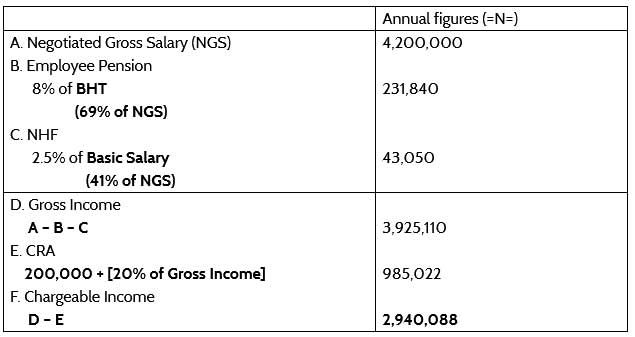Upturn

# How to calculate graduated taxes

## - Calculating Pay-As-You-Earn Tax

Drag to resize
So you want to calculate taxes, innit? Well, read on then.

The first thing to know about how to calculate tax (Pay-As-You-Earn tax) is that it is levied on the employee's chargeable income and calculated at graduated rates. The Sixth Schedule, Section 37(3) of the Amended Personal Income Tax Act, 2011 determines the rates at which tax is to be graduated, and the schedule is given as:
Tax Income Rates:
• First 300,000 to be taxed at 7%
• Next 300,000 to be taxed at 11%
• Next 500,000 to be taxed at 15%
• Next 500,000 to be taxed at 19%
• Next 1,600,000 to be taxed at 21%
• Next 3,200,000 to be taxed at 24%
Now, pay attention. What these graduated tax rates mean is that tax is calculated on parts of the chargeable income at specific, compounding percentages. So the first 300,000 out of an employee's ANNUAL chargeable income is taxed at 7%, then the next 300,000 is taxed at 11%. The next 500,000 after that is taxed at 15%, and so on and so forth, until there is no chargeable income left.

These graduated rates are then summed up and deducted from the gross income to arrive at Net Salary.

Let's use an example to illustrate:

Enter Rob, an employee at ABC Ltd.  Rob negotiated a gross salary of 350,000, which gives his annual gross figure as 4,200,000. Let's go all the way from Rob's negotiated gross salary to his chargeable income, in order to calculate his taxes.

The table below would be the natural sequence of things on the payroll for ABC Ltd, under Rob's name. If you want to understand why we deducted what we did, read this.Now, it is on this amount, 2,940,088 that tax will be levied at the graduated rates we had earlier discussed.

Let's start with the first stage: first 300,000 at 7%. This gives:
7% x 300,000 = 21,000

Next, we have 11% on the next 300,000. That gives:
11% x 300,000 = 33,000

For the next 500,000, we have 15% on that, which gives:
15% x 500,000 = 75,000

Next is 19% on the next 500,000, which gives:
19% x 500,000 = 95,000

Right after that, we should calculate 21% on the next 1,600,000. But this will not be possible because we cannot get a full 1,600,000 out of what's left of Rob's chargeable income. What we have left is 1,340,088, so we'll just go ahead to calculate 21% on that.

21% x 1,340,088 = 281,418.48

The reason is this: if you haven't noticed by now, after calculating each percentage, you need to make sure that the next amount can be extracted from what's left of the chargeable income to calculate the next percentage on.

For example, when we calculated 7% on the first 300,000 from the initial chargeable income of 2,940,088, we would have had 2640,088 left to calculate 11% on the next 300,000 from it, which would have left us with 2,340,088, on which to calculate 15% on the next 500,000... and you get the idea.

So by the time we had removed the first 300,000, the next 300,000, the next 500,000, and the next 500,000, we didn't have up to 1,600,000 left, and so we just calculated our 21% on what was left and moved on.

So if we had another employee with a lesser salary who, let's say, we would not be able get the first 500,000 out of, we would simply calculate 15% on what's left, after we might have calculated 7% and 11% on the first and second 300,000 respectively.

Get my drift?

So for Rob, we won't even get up to the last 3,200,000 to calculate 24% on, so we'll stop here.

What we'll do next is to sum up the results of these graduated rates and deduct the total figure from his negotiated gross salary.

That would give us:
21,000 + 33,000 + 75,000 + 95,000 + 281,418.48 = 505,418.98 as Rob's annual tax.

If we divide that by 12, we get 42,118.21 as his monthly tax.

Going forward, his monthly net salary would then be his gross income minus this tax. If you remember, his annual gross income was 3,925,110 from the table. Divide that by 12 and you get 327,092.50 as his monthly gross income.

So his Net Salary would be:
327,092.50 - 42,118 = 284,974.29 per month.

That was a lot, I know. But I have a better way.

(You don't mean it!)

Yup. With our Payroll course, you can do away with the long explanation that has been this blog and learn a practical way to calculate your taxes on your payroll. What's more, you get a free Payroll template, complete with a PAYE tax calculator that computes your graduated taxes, even sums them up, and deducts them from your gross income to arrive at your net salary!

Amazing, right???

So what do you say? You want to go over this blog again to try to master how to calculate graduated taxes manually, or would you rather save yourself some trouble and go with our payroll tax calculator?

Make the best decision by clicking the shiny teal button below. Do it now!
Drag to resize